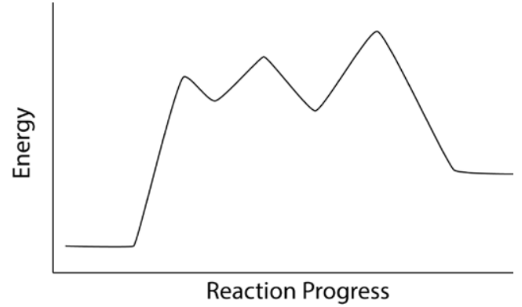# Problem: Which of the following statements pertaining to the reaction coordinate plot below is/are correct.I. The overall reaction is endothermicII. The overall reaction is exothermicIII. There is a transition stateIV. There is one intermediateV. There are two intermediatesA. Only statements I and III are correctB. Only statements I and IV are correctC. Only statements I and V are correctD. Only statements II and III are correctE. Only statements II and IV are correct

###### FREE Expert Solution

We’re being asked to which of the following statements are true based on the energy diagram.

Recall that an energy diagram is usually read from left to right

The components of an energy diagram are:

• Reactants: are placed on the left/beginning of the energy diagram

• Products: are placed on the right/end of the energy diagram

• transition states: are the peaks in the energy diagram

• Intermediates: are formed in-between steps or the momentary dips in the energy diagram

• Energy change (ΔH or ΔG˚): is the difference in energy between products and reactants

• When the energy of the product is lower than the reactant then it is exothermic.
• When the energy of the product is higher than the reactant then it is endothermic.

Activation energy (Ea): is the difference in energy between reactants and transition state###### Problem Details

Which of the following statements pertaining to the reaction coordinate plot below is/are correct.

I. The overall reaction is endothermic

II. The overall reaction is exothermic

III. There is a transition state

IV. There is one intermediate

V. There are two intermediatesA. Only statements I and III are correct

B. Only statements I and IV are correct

C. Only statements I and V are correct

D. Only statements II and III are correct

E. Only statements II and IV are correct